The TAC is the average historical catch over the last yrsmth (default 5) years, multiplied by (1-xx)

CC1(x, Data, reps = 100, plot = FALSE, yrsmth = 5, xx = 0)

CC2(x, Data, reps = 100, plot = FALSE, yrsmth = 5, xx = 0.1)

CC3(x, Data, reps = 100, plot = FALSE, yrsmth = 5, xx = 0.2)

CC4(x, Data, reps = 100, plot = FALSE, yrsmth = 5, xx = 0.3)

CC5(x, Data, reps = 100, plot = FALSE, yrsmth = 5, xx = 0.4)

CurC(x, Data, reps = 100, plot = FALSE, yrsmth = 1, xx = 0)

## Arguments

x

A position in the data object

Data

A data object

reps

The number of stochastic samples of the MP recommendation(s)

plot

Logical. Show the plot?

yrsmth

Years over which to calculate mean catches

xx

Parameter controlling the TAC. Mean catches are multiplied by (1-xx)

## Value

An object of class Rec-class with the TAC slot populated with a numeric vector of length reps

## Details

The TAC is calculated as: $$\textrm{TAC} = (1-x)C_{\textrm{ave}}$$ where x lies between 0 and 1, and $$C_{\textrm{ave}}$$ is average historical catch over the previous yrsmth years.

The TAC is constant for all future projections.

## Functions

• CC1: TAC is average historical catch from recent yrsmth years

• CC2: TAC is average historical catch from recent yrsmth years reduced by 10\

• CC3: TAC is average historical catch from recent yrsmth years reduced by 20\

• CC4: TAC is average historical catch from recent yrsmth years reduced by 30\

• CC5: TAC is average historical catch from recent yrsmth years reduced by 40\

• CurC: TAC is fixed at last historical catch

## Required Data

See Data-class for information on the Data object

CC1: Cat, LHYear, Year

## Rendered Equations

See Online Documentation for correctly rendered equations

Other Constant Catch MPs: GB_CC()

T. Carruthers

## Examples

CC1(1, MSEtool::Cobia, plot=TRUE)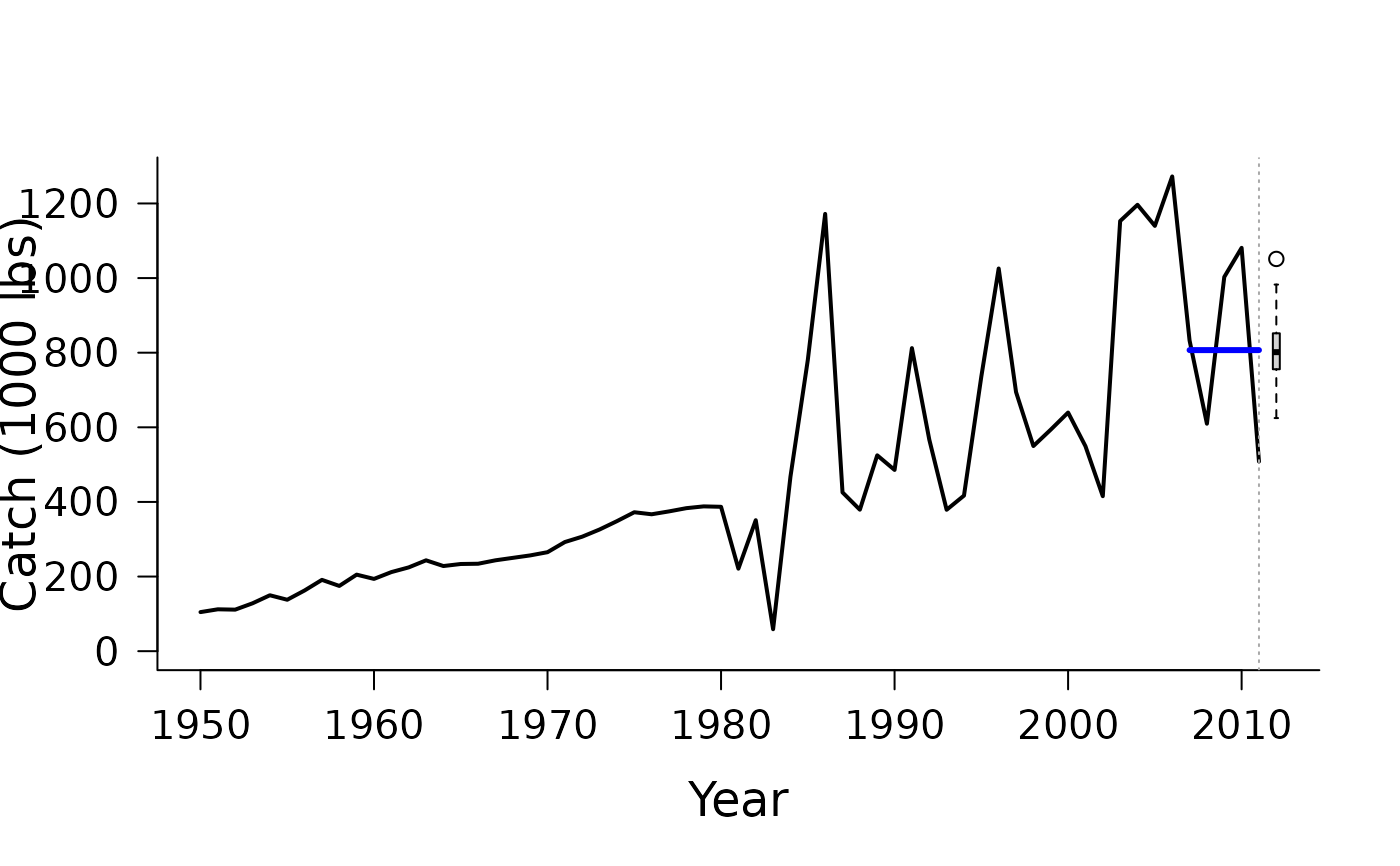#> TAC (median)
#>     797.6159

CC2(1, MSEtool::Cobia, plot=TRUE)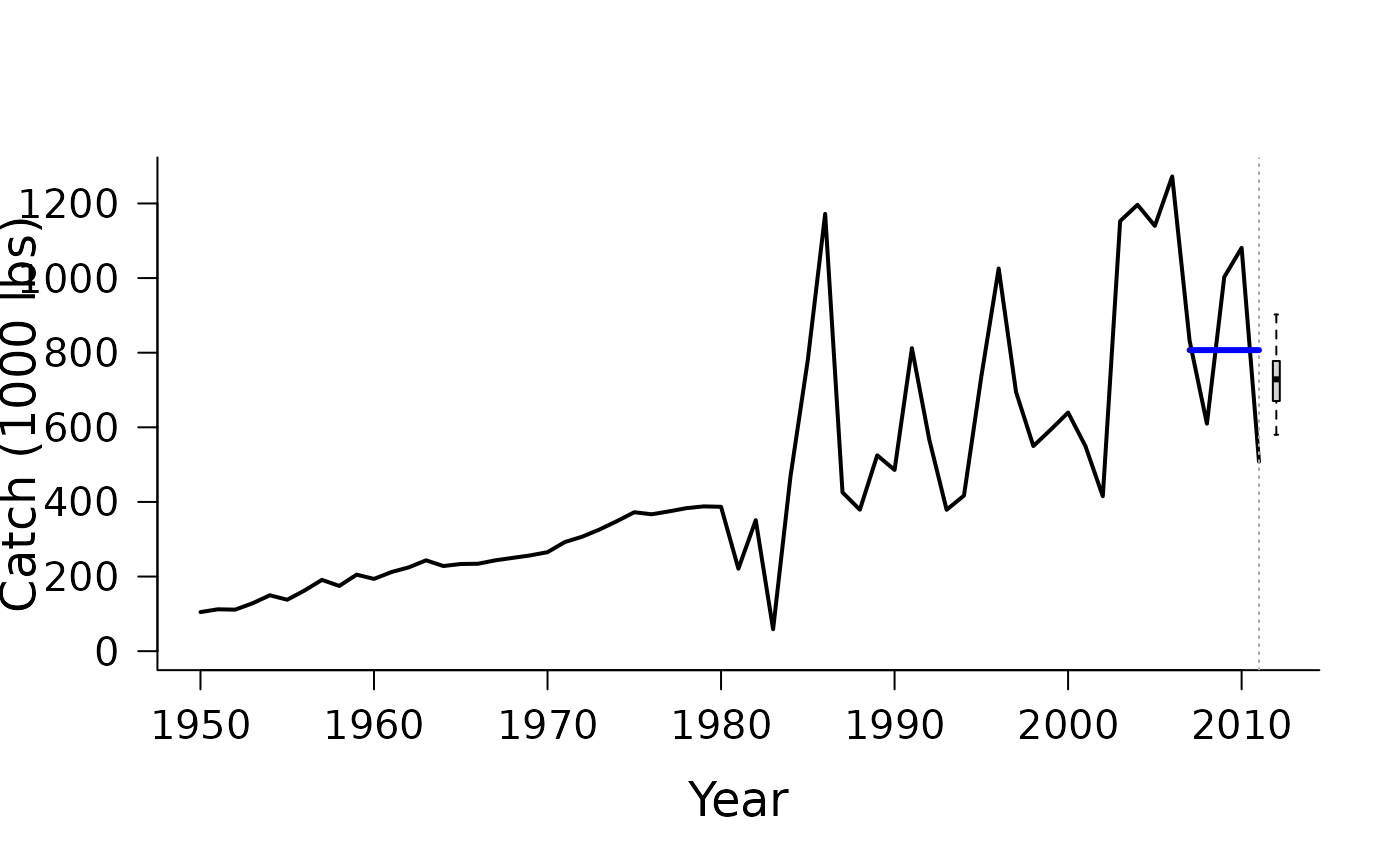#> TAC (median)
#>     716.9905

CC3(1, MSEtool::Cobia, plot=TRUE)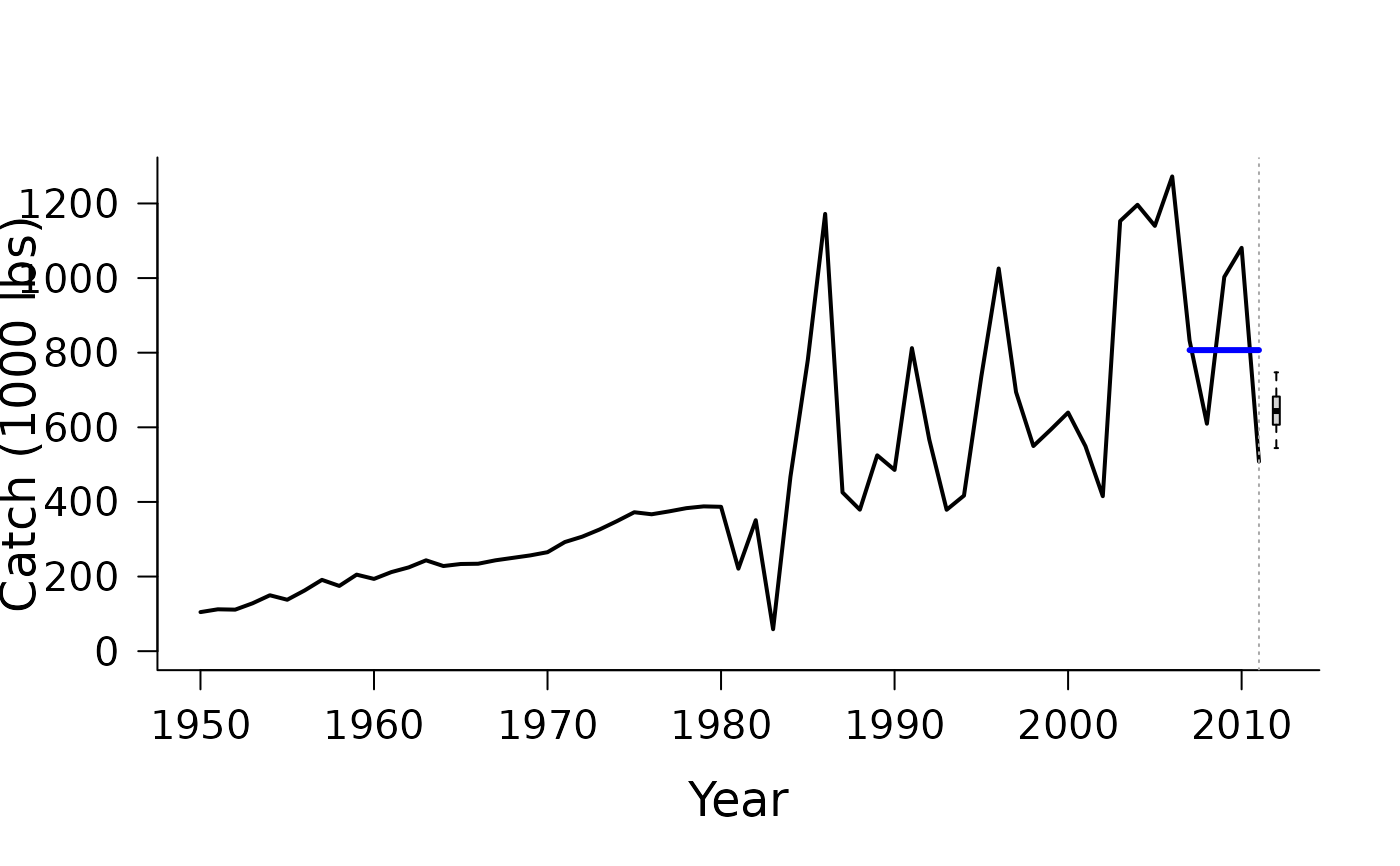#> TAC (median)
#>     644.0886

CC4(1, MSEtool::Cobia, plot=TRUE)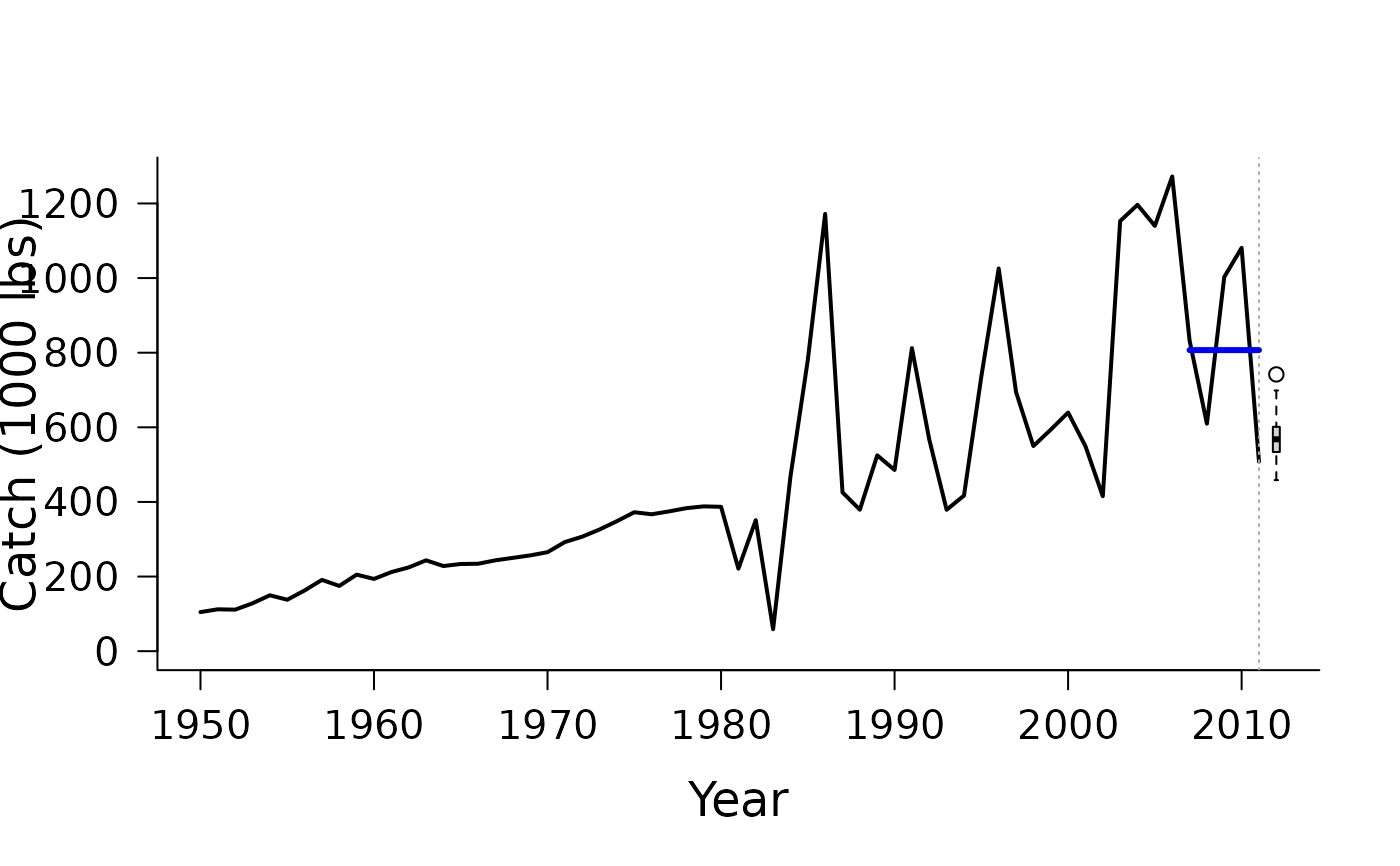#> TAC (median)
#>     558.6875

CC5(1, MSEtool::Cobia, plot=TRUE)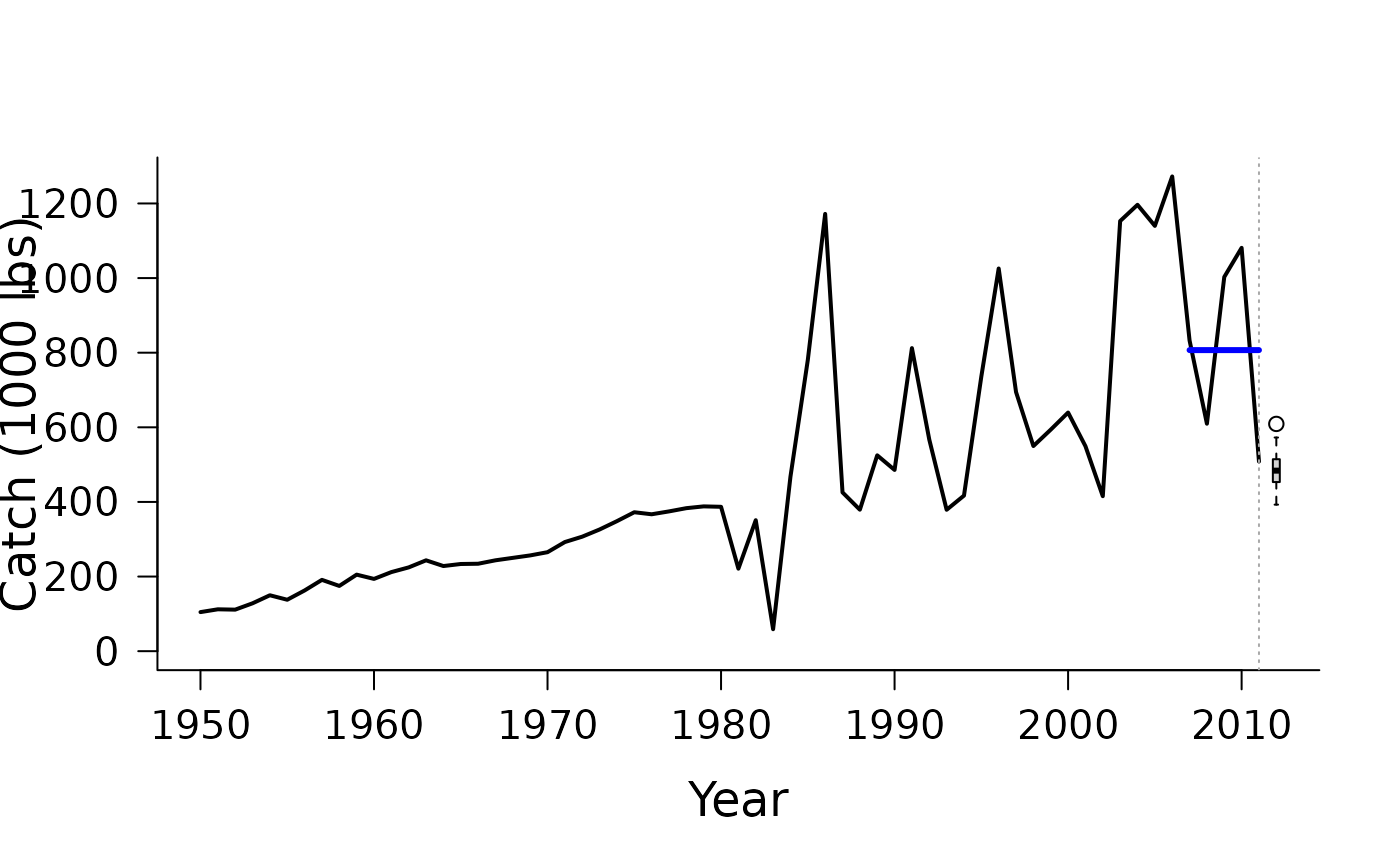#> TAC (median)
#>     474.7955

CurC(1, MSEtool::Cobia, plot=TRUE)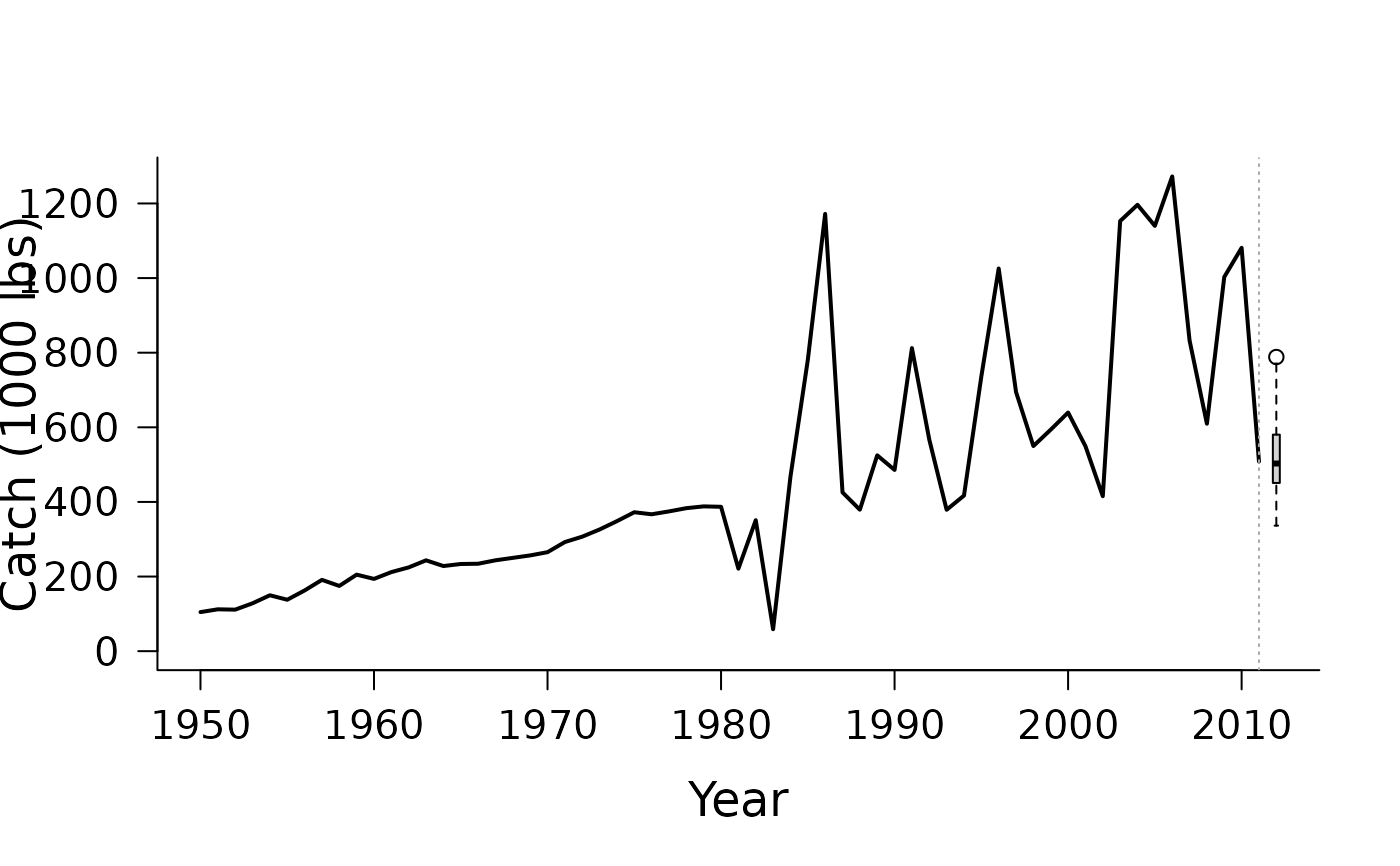#> TAC (median)
#>     492.3154